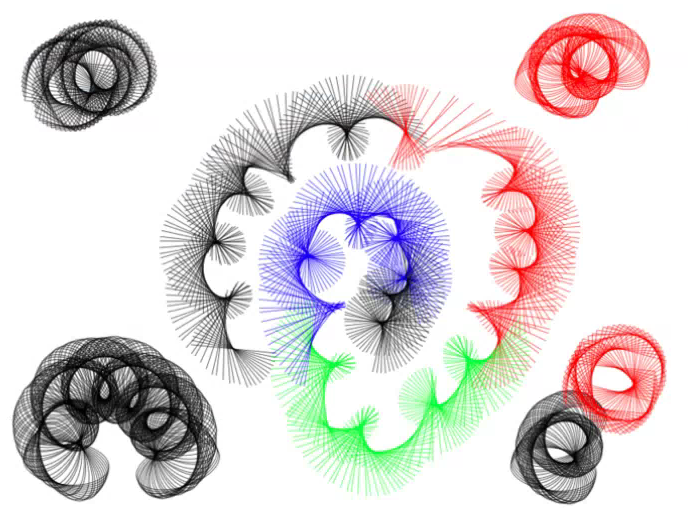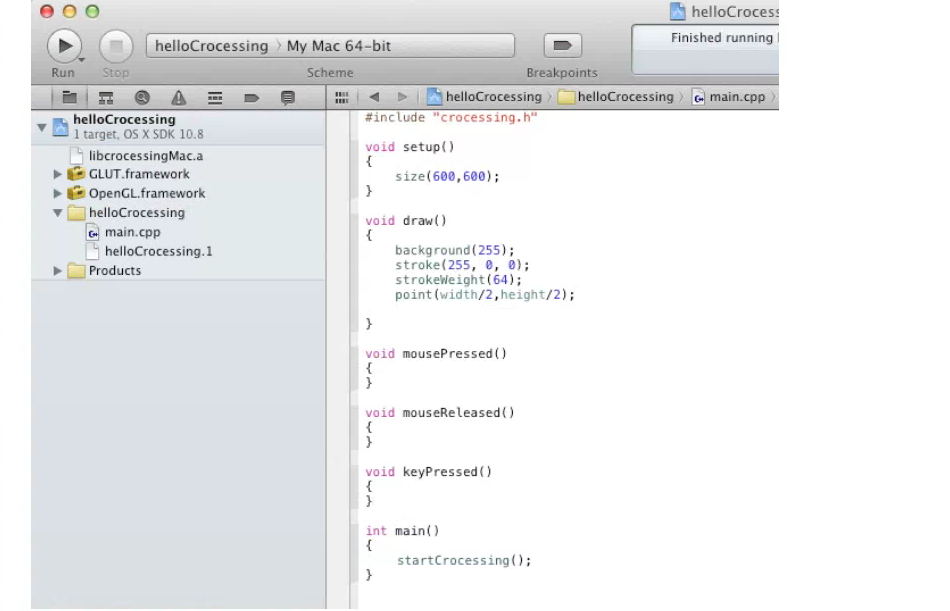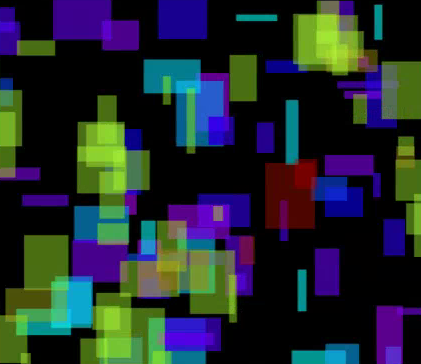# Crocessing | Library for Processing Style Programming in C Language.

Processing provides an easy way to program for interactive visual applications, but is slow in performance. C is fast in performance, but difficult to program. "Crocessing" aims to fill the gap. It is a C library that allows code written in Processing to run as fast as native C code with minimal changes.Supported Features:

// User Input handling functions

void mousePressed();

void mouseReleased();

void mouseMoved();

void mouseDragged();

void keyPressed();

// Utility Functions

float constrain(float in, float min, float max);

float dist(float x1, float y1, float x2, float y2);

float map(float in, float in_min, float in_max, float out_min, float out_max);

void frameRate(int in);

float radians(float in);

int millis();

float min(float a, float b);

float max(float a, float b);

void setup();

void draw();

void size(int x, int y, int mode);

void size(int x, int y);

void random(float s, float e);

// Audio Loading and Playing

CAudio loadAudio(char *name);

void playAudio(CAudio in, float volume=1.f);

// Image Loading and Displaying

CImage loadImage(const char *filename);

void image(CImage image, int x, int y);

void image(CImage image, int x, int y, int w, int h);

void imageMode(_ALIGN_MODE mode);

// 2D/3D Graphics functions

void beginShape(_SHAPE_MODE mode=LINE_STRIP);

void endShape(_SHAPE_MODE mode = NA);

void vertex(float x, float y);

void vertex(float x, float y, float z);

void vertex(float x, float y, float u, float v);

void vertex(float x, float y, float z, float u, float v);

void texture(CImage img);

void noFill();

void noStroke();

void translate(float x, float y);

void rotate(float th);

void ellipse(int x, int y, int width, int height);

void fill(float a, float b, float c, float d);

void fill(float a, float b, float c);

void fill(float a);

void rect(int x, int y, int w, int h);

void strokeWeight(float in);

void line(int x1, int y1, int x2, int y2);

void background(float p1, float p2, float p3, float p4);

void background(float p1, float p2, float p3);

void background(float p1);

void stroke(float p1, float p2, float p3, float p4);

void stroke(float p1, float p2, float p3);

void stroke(float p1);

void point(int x, int y);

void rect(int x, int y, int w, int h);

void colorMode(_COLOR_MODE mode);

// Text Functions

void text(const char *text, int x, int y);

void textSize(int size);

* Crocessing was initially developed for C language class by Jusub Kim at Sogang University in 2013 and was also used successfully for a mobile UI development project with LG Electronics in 2013

* If you are interested in Crocessing, you can download source code at